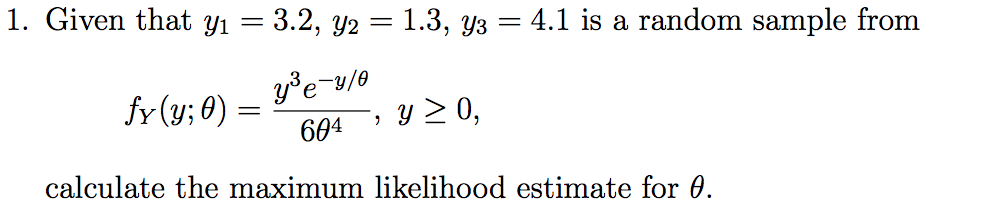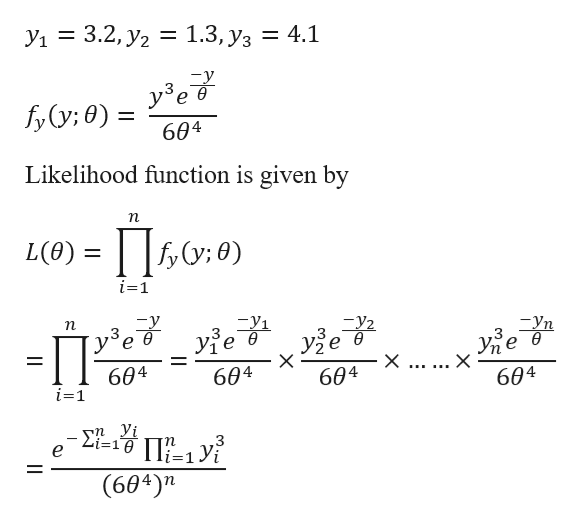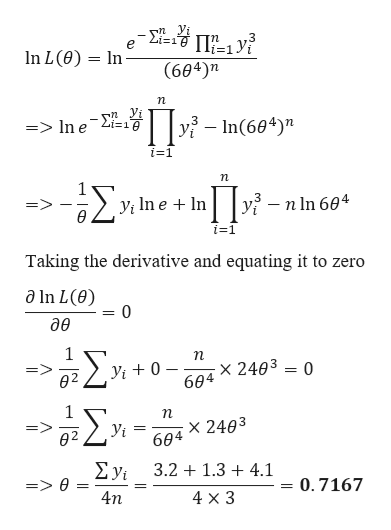# 1. Given that yı = 3.2, y2 = 1.3, y3 = 4.1 is a random sample fromyeu/ofr (y;0)=604y 20,calculate the maximum likelihood estimate for 0.

Question
28 viewshelp_outlineImage Transcriptionclose1. Given that yı = 3.2, y2 = 1.3, y3 = 4.1 is a random sample from yeu/o fr (y;0)= 604 y 20, calculate the maximum likelihood estimate for 0. fullscreen
check_circle

Step 1

Given datahelp_outlineImage Transcriptionclose= 3.2, y2 1.3, y3 = 1.3, Уз = 4.1 y ев fyy: 0) 11 604 Likelihood function is given by п L(),: 0) O:0) i=1 n -y1 y2 — Уп 3 ө узе в ује в X ... ... X 604 604 604 604 i=1 -i=1& |=1 Yi е ,3 (604)n fullscreen
Step 2

Taking natural Log...help_outlineImage Transcriptionclose- Σ In L (e) In (604)n yi-In(604)" => In e 2i=19 i=1 n Σ yi n ln 604 i=1 Taking the derivative and equating it to zero a In L(e) 0 дө Σ 1 п x 2403 0 604 yi 0 02 Σ x 2403 604 => ө2 У Σν 3.2 1.34.1 0.7167 4n 4 x 3 fullscreen

### Want to see the full answer?

See Solution

#### Want to see this answer and more?

Solutions are written by subject experts who are available 24/7. Questions are typically answered within 1 hour.*

See Solution
*Response times may vary by subject and question.
Tagged in

### Other